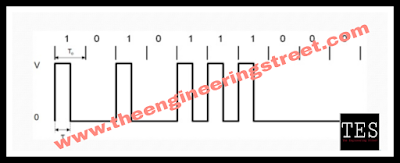# What Is Line Coding And Classification Of Line Codes??

## What Is Line Coding And Classification Of Line Codes??

Classification Of Lines :

### Line Coding

The line coding is defined as the process of converting binary data,a sequence of bits to a digital signal.

The digital data such as text, numbers,graphical images,audio and video are stored in computer memory in the form of sequences of bits.

Line coding converts these sequence into digital signal as shown in figure.Line Coding

### Classification of Line Codes:Classification of line coding

The line codes are basically divided into three categories:
1. Unipolar codes
2. Polar codes
3. Bipolar codes

#### 1. Unipolar codes:

• Unipolar codes use only one voltage level other than zero.
• So the encoded signal will have either +A volts value or 0.
• These codes are very simple and primitive and are not used now a days.
• Unipolar has two formats RZ and NRZ unipolar format

#### 2. Polar codes:

• Polar coding using two voltage levels other than zero such as +A/2 and -A/2 volts.
• This will bring the dc level for some codes to zero which is a desired characteristics.
• Polar has RZ&NRZ polar format and Manchester format.

#### 3. Bipolar codes:

• Bipolar coding uses three voltage level positive,negative and zero which is similar to polar codes.
• But here the zero level is always used for representing the "0" in the data stream at the input.
• Bipolar has Bipolar AMI format.

### Unipolar RZ Formate:Unipolar RZ format

The return to zero (RZ) unipolar format is as shown in Figure.

In this format every "0" is represented by an off pulse (0) and each "1" by an on pulse with amplitude A and a duration of T / 2, followed by a return to zero level.

Therefore this is Called as return to zero (RZ) format As the voltage level is either + A or zero, this is a unipolar format (Unipolar means only one polarity).

Due to the unipolar nature, the unipolar RZ format has a nonzero de value. The dc value does act contain any information.

### Unipolar NRZ Formate:Unipolar NRZ format

A non return  to zero (NRZ) format is as shown in Figure.

In this format a logic "1" is represented by a pulse of full bit duration Tb and amplitude + A while a logic "0" is represented by an off pulse or zero amplitude.

During the on time, the pulse does not return to zero after half bit period.

Therefore the name NRZ format. As the pulses have either + A or 0 amplitude it is called as a unipolar format.

Internal computer waveforms are usually of this type. Due to the unipolar nature, the unipolar NRZ format also will have a nonzero average (dc) value which does not carry any information.

Due to longer pulse duration, the NRZ pulses carry "energy" than the RZ pulses.

But they need synchronization at the receiver as there is no separation between the adjacent pulses

### Polar RZ Format:Polar RZ format

The disadvantage of the two unipolar formats discussed earlier is that they result in a dc component that does not carry any information and wastes power.

The polar RZ format is as shown in Figure. It shows that opposite polarity pulses of amplitude "+A/2 or - A/2 " are used to represent logic "1" and "0".

Therefore it is called as a "polar" format.

As the pulses return to zero after half the bit duration T/2 this format is a RZ format.

### Polar NRZ Format:Polar NRZ format

In the polar NRZ format, as shown in figure a pulse of amplitude "+ A/2" of duration Tb, is used to represent a logic "1" and a pulse of amplitude - A/2" of the same duration represents a logic "0"

Unlike the unipolar waveform, a polar waveform has no dc component if the 0s and ls in the input data occur in equal proportion.

### Bipolar NRZ Format(AMI):Bipolar NRZ format

The bipolar NRZ format is as shown in Fig.

Here the successive "1s" are represented pulses with alternating polarity, and no pulse is transmitted for a logic "0".

Note that in this representation there are three levels : + A, 0 and - A Therefore this is also known as "pseudoternary or Alternative Mark Inversion (AMI)" format.

An attractive feature of the bipolar format is the absence of a dc component eventhough the input  binary data may contains long strings of 0s" and "1s.

Moreover the bipolar format eliminates ambiguity that may arise because of polarity inversion during the course of transmissions. (This problem is observed in the switched telephone networks).

This is the reason why the bipolar NRZ format is used in the PCM-TDM T1, system for telephony.

The absence of dc component allows the use of transformers for coupling.

### Split phase Manchester Format:Manchester format

The split phase Manchester format shown in figure.

In this format symbol 1 represented by transmitting a positive pulse of "+A/2" amplitude for one half of the symbol duration, followed by  a negative pulse amplitude "- A/2" for remaining half of the symbol duration.

For symbol "0" these two phase are transmitted in reverse order.

This waveform does not have any dc component.

The Manchester format has a built in synchronization capability as it crosses zero at regular interval.

But this capability is attained at the expense of a bandwidth requirement of twice that of the NRZ unipolar , polar and bipolar.

Local Area Networks (LAN) such a Ethernet and Cheapest are increasing using the Manchester code for signal transmission over the network.

May You Like To Read This More Related Informative Articles On Electronics Engineering :